# Tag: Spivak

Brendan Fong, David Spivak and Remy Tuyeras cooked up a vast generalisation of neural networks in their paper Backprop as Functor: A compositional perspective on supervised learning.

Here’s a nice introduction to neural networks for category theorists by Bruno Gavranovic. At 1.49m he tries to explain supervised learning with neural networks in one slide. Learners show up later in the talk.

$\mathbf{Poly}$ is the category of all polynomial functors, that is, things of the form
$p = \sum_{i \in p(1)} y^{p[i]}~:~\mathbf{Sets} \rightarrow \mathbf{Sets} \qquad S \mapsto \bigsqcup_{i \in p(1)} Maps(p[i],S)$
with $p(1)$ and all $p[i]$ sets.

Last time I gave Spivak’s ‘corolla’ picture to think about such functors.

I prefer to view $p \in \mathbf{Poly}$ as an horribly discrete ‘sheaf’ $\mathcal{P}$ over the ‘space’ $p(1)$ with stalk $p[i]=\mathcal{P}_i$ at point $i \in p(1)$.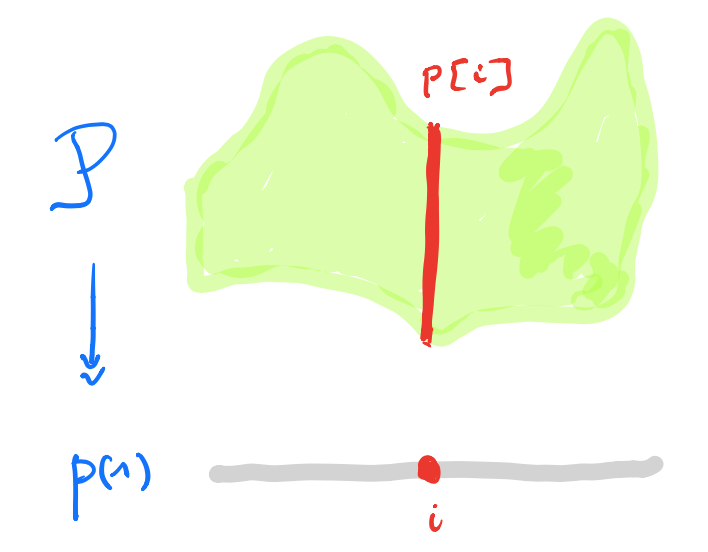A morphism $p \rightarrow q$ in $\mathbf{Poly}$ is a map $\varphi_1 : p(1) \rightarrow q(1)$, together with for all $i \in p(1)$ a map $\varphi^{\#}_i : q[\varphi_1(i)] \rightarrow p[i]$.

In the sheaf picture, this gives a map of sheaves over the space $p(1)$ from the inverse image sheaf $\varphi_1^* \mathcal{Q}$ to $\mathcal{P}$.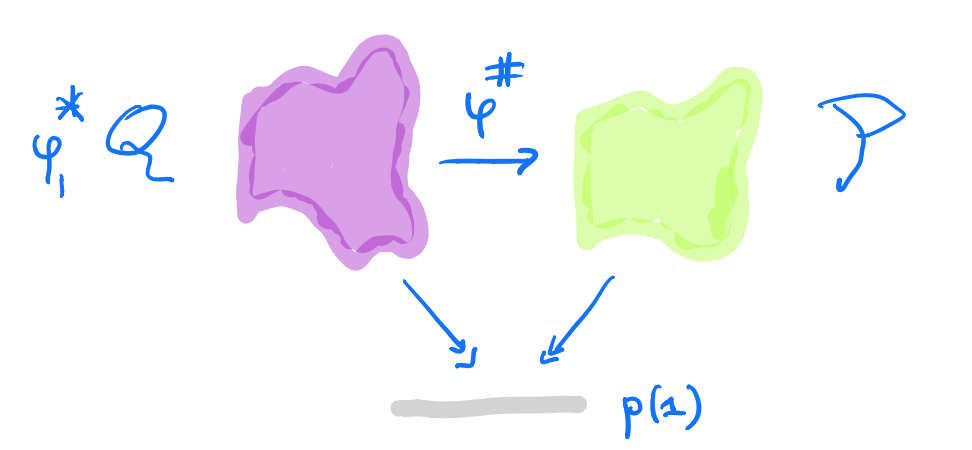But, unless you dream of sheaves in the night, by all means stick to Spivak’s corolla picture.

A learner $A \rightarrow B$ between two sets $A$ and $B$ is a complicated tuple of things $(P,I,U,R)$:

• $P$ is a set, a parameter space of some maps from $A$ to $B$.
• $I$ is the interpretation map $I : P \times A \rightarrow B$ describing the maps in $P$.
• $U$ is the update map $U : P \times A \times B \rightarrow P$, the learning procedure. The idea is that $U(p,a,b)$ is a map which sends $a$ closer to $b$ than the map $p$ did.
• $R$ is the request map $R : P \times A \times B \rightarrow A$.

Here’s a nice application of $\mathbf{Poly}$’s set-up:

Morphisms $\mathbf{P y^P \rightarrow Maps(A,B) \times Maps(A \times B,A) y^{A \times B}}$ in $\mathbf{Poly}$ coincide with learners $\mathbf{A \rightarrow B}$ with parameter space $\mathbf{P}$.

This follows from unpacking the definition of morphism in $\mathbf{Poly}$ and the process CT-ers prefer to call Currying.

The space-map $\varphi_1 : P \rightarrow Maps(A,B) \times Maps(A \times B,A)$ gives us the interpretation and request-map, whereas the sheaf-map $\varphi^{\#}$ gives us the more mysterious update-map $P \times A \times B \rightarrow P$.

$\mathbf{Learn(A,B)}$ is the category with objects all the learners $A \rightarrow B$ (for all paramater-sets $P$), and with morphisms defined naturally, that is, maps between the parameter-sets, compatible with the structural maps.

A surprising result from David Spivak’s paper Learners’ Languages is

$\mathbf{Learn(A,B)}$ is a topos. In fact, it is the topos of all set-valued representations of a (huge) directed graph $\mathbf{G_{AB}}$.

This will take some time.

Let’s bring some dynamics in. Take any polynmial functor $p \in \mathbf{Poly}$ and fix a morphism in $\mathbf{Poly}$
$\varphi = (\varphi_1,\varphi[-])~:~p(1) y^{p(1)} \rightarrow p$
with space-map $\varphi_1$ the identity map.

We form a directed graph:

• the vertices are the elements of $p(1)$,
• vertex $i \in p(1)$ is the source vertex of exactly one arrow for every $a \in p[i]$,
• the target vertex of that arrow is the vertex $\phi[i](a) \in p(1)$.

Here’s one possibility from Spivak’s paper for $p = 2y^2 + 1$, with the coefficient $2$-set $\{ \text{green dot, yellow dot} \}$, and with $1$ the singleton $\{ \text{red dot} \}$.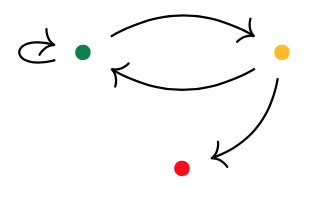Start at one vertex and move after a minute along a directed edge to the next (possibly the same) vertex. The potential evolutions in time will then form a tree, with each node given a label in $p(1)$.

If we start at the green dot, we get this tree of potential time-evolutions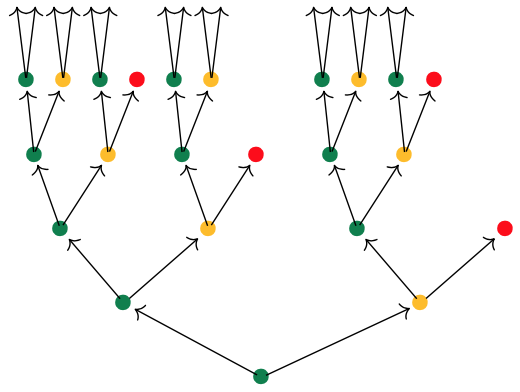There are exactly $\# p[i]$ branches leaving a node labeled $i \in p(1)$, and all subtrees emanating from equal labelled nodes are isomorphic.

If we had started at the yellow dot we had obtained a labelled tree isomorphic to the subtree emanating here from any yellow dot.

We can do the same things for any morphism in $\mathbf{Poly}$ of the form
$\varphi = (\varphi_1,\varphi[-])~:~Sy^S \rightarrow p$
Now, we have a directed graph with vertices the elements $s \in S$, with as many edges leaving vertex $s$ as there are elements $a \in p[\varphi_1(s)]$, and with the target vertex of the edge labeled $a$ starting in $s$ the vertex $\varphi[\varphi_1(s)](A)$.

Once we have this directed graph on $\# S$ vertices we can label vertex $s$ with the label $\varphi_1(s)$ from $p(1)$.

In this way, the time evolutions starting at a vertex $s \in S$ will give us a $p(1)$-labelled rooted tree.

But now, it is possibly that two distinct vertices can have the same $p(1)$-labeled tree of evolutions. But also, trees corresponding to equal labeled vertices can be different.

Right, I guess we’re ready to define the graph $G_{AB}$ and prove that $\mathbf{Learn(A,B)}$ is a topos.

In the case of learners, we have the target polynomial functor $p=C y^{A \times B}$ with $C = Maps(A,B) \times Maps(A \times B,A)$, that is
$p(1) = C \quad \text{and all} \quad p[i]=A \times B$

Start with the free rooted tree $T$ having exactly $\# A \times B$ branches growing from each node.

Here’s the directed graph $G_{AB}$:

• vertices $v_{\chi}$ correspond to the different $C$-labelings of $T$, one $C$-labeled rooted tree $T_{\chi}$ for every map $\chi : vtx(T) \rightarrow C$,
• arrows $v_{\chi} \rightarrow v_{\omega}$ if and only if $T_{\omega}$ is the rooted $C$-labelled tree isomorphic to the subtree of $T_{\chi}$ rooted at one step from the root.

A learner $\mathbf{A \rightarrow B}$ gives a set-valued representation of $\mathbf{G_{AB}}$.

We saw that a learner $A \rightarrow B$ is the same thing as a morphism in $\mathbf{Poly}$
$\varphi = (\varphi_1,\varphi[-])~:~P y^P \rightarrow C y^{A \times B}$
with $P$ the parameter set of maps.

Here’s what we have to do:

1. Draw the directed graph on vertices $p \in P$ giving the dynamics of the morphism $\varphi$. This graph describes how the learner can cycle through the parameter-set.

2. Use the map $\varphi_1$ to label the vertices with elements from $C$.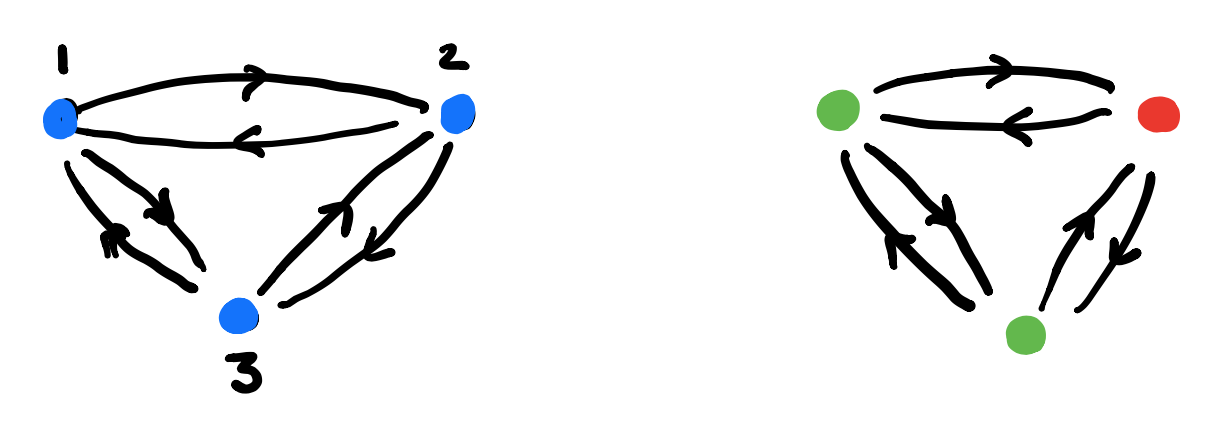3. For each vertex draw the rooted $C$-labeled tree of potential time-evolutions starting in that vertex.

In this example the time-evolutions of the two green vertices are the same, but in general they can be different.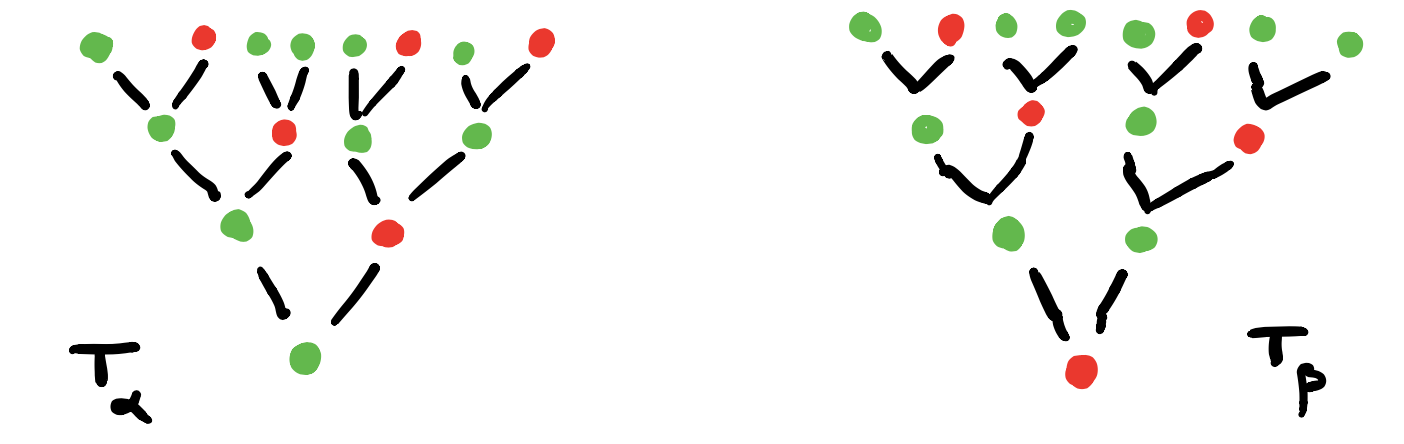4. Find the vertices in $G_{AB}$ determined by these $C$-labeled trees and note that they span a full subgraph of $G_{AB}$.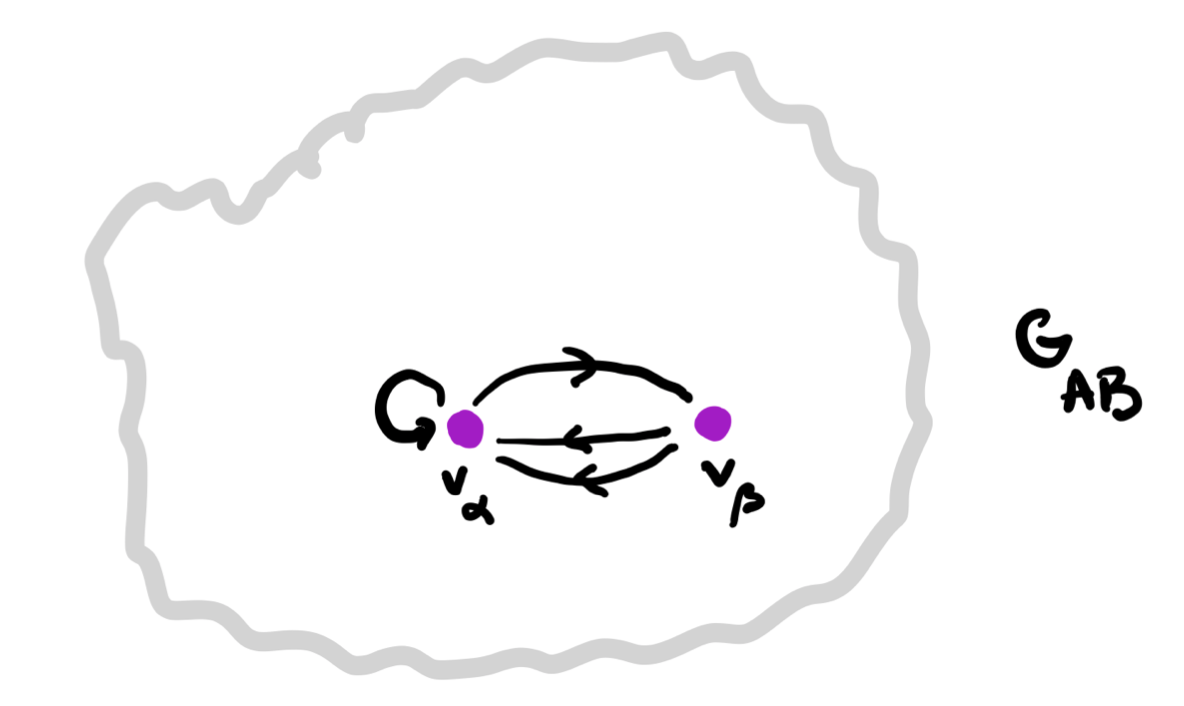5. The vertex-set $P_v$ consists of all elements from $p$ whose ($C$-labeled) vertex has evolution-tree $T_v$. If $v \rightarrow w$ is a directed edge in $G_{AB}$ corresponding to an element $(a,b) \in A \times B$, then the map on the vertex-sets corresponding to this edge is
$f_{v,(a,b)}~:~P_v \rightarrow P_w \qquad p \mapsto \varphi[\varphi_1(p)](a,b)$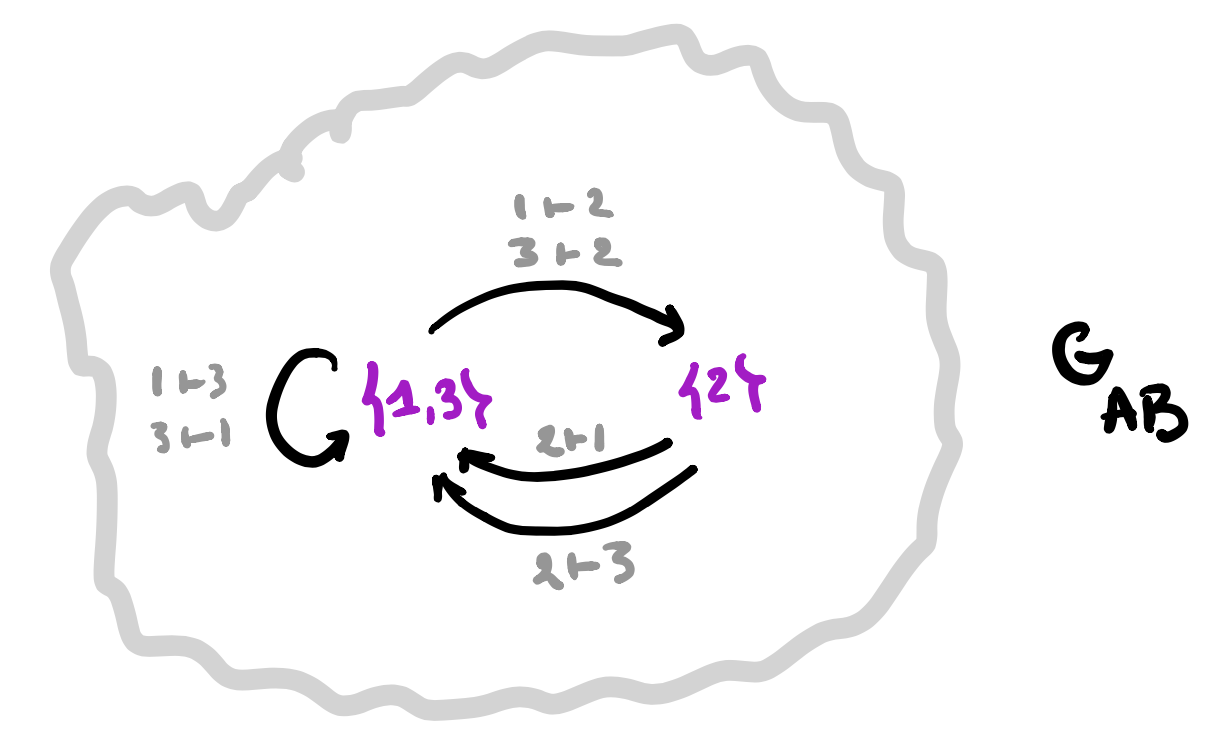A set-valued representation of $\mathbf{G_{AB}}$ gives a learner $\mathbf{A \rightarrow B}$.

1. Take a set-valued representation of $G_{AB}$, that is, the finite or infinite collection of vertices $V$ in $G_{AB}$ where the vertex-set $P_v$ is non-empty. Note that these vertices span a full subgraph of $G_{AB}$.

And, for each directed arrow $v \rightarrow w$ in this subgraph, labeled by an element $(a,b) \in A \times B$ we have a map
$f_{v,(a,b)}~:~P_v \rightarrow P_w$

2. The parameter set of our learner will be $P = \sqcup_v P_v$, the disjoint union of the non-empty vertex-sets.

3. The space-map $\varphi_1 : P \rightarrow C$ will send an element in $P_v$ to the $C$-label of the root of the tree $T_v$. This gives us already the interpretation and request maps
$I : P \times A \rightarrow B \quad \text{and} \quad R : P \times A \times B \rightarrow A$

4. The update map $U : P \times A \times B \rightarrow P$ follows from the sheaf-map we can define stalk-wise
$\varphi[\varphi_1(p)](a,b) = f_{v,(a,b)}(p)$
if $p \in P_v$.

That’s all folks!

$\mathbf{Learn(A,B)}$ is equivalent to the (covariant) functors $\mathbf{G_{AB} \rightarrow Sets}$.

Changing the directions of all arrows in $G_{AB}$ any covariant functor $\mathbf{G_{AB} \rightarrow Sets}$ becomes a contravariant functor $\mathbf{G_{AB}^o \rightarrow Sets}$, making $\mathbf{Learn(A,B)}$ an honest to Groth topos!

Every topos comes with its own logic, so we have a ‘learners’ logic’. (to be continued)

Following up on the deep learning and toposes-post, I was planning to do something on the logic of neural networks.

Prepping for this I saw David Spivak’s paper Learner’s Languages doing exactly that, but in the more general setting of ‘learners’ (see also the deep learning post).

And then … I fell under the spell of $\mathbf{Poly}$.

Spivak is a story-telling talent. A long time ago I copied his short story (actually his abstract for a talk) “Presheaf, the cobbler” in the Children have always loved colimits-post.

Last week, he did post Poly makes me happy and smart on the blog of the Topos Institute, which is another great read.

If this is way too ‘fluffy’ for you, perhaps you should watch his talk Poly: a category of remarkable abundance.

If you like (applied) category theory and have some days to waste, you can binge-watch all 15 episodes of the Poly-course Polynomial Functors: A General Theory of Interaction.

If you are more the reading-type, the 273 pages of the Poly-book will also kill a good number of your living hours.

Personally, I have no great appetite for category theory, I prefer to digest it in homeopathic doses. And, I’m allergic to co-terminology.

So then, how to define $\mathbf{Poly}$ for the likes of me?

$\mathbf{Poly}$, you might have surmised, is a category. So, we need ‘objects’ and ‘morphisms’ between them.

Any set $A$ has a corresponding ‘representable functor’ sending a given set $S$ to the set of all maps from $A$ to $S$
$y^A~:~\mathbf{Sets} \rightarrow \mathbf{Sets} \qquad S \mapsto S^A=Maps(A,S)$
This looks like a monomial in a variable $y$ ($y$ for Yoneda, of course), but does it work?

What is $y^1$, where $1$ stands for the one-element set $\{ \ast \}$? $Maps(1,S)=S$, so $y^1$ is the identity functor sending $S$ to $S$.

What is $y^0$, where $0$ is the empty set $\emptyset$? Well, for any set $S$ there is just one map $\emptyset \rightarrow S$, so $y^0$ is the constant functor sending any set $S$ to $1$. That is, $y^0=1$.

Going from monomials to polynomials we need an addition. We add such representable functors by taking disjoint unions (finite or infinite), that is
$\sum_{i \in I} y^{A_i}~:~\mathbf{Sets} \rightarrow \mathbf{Sets} \qquad S \mapsto \bigsqcup_{i \in I} Maps(A_i,S)$
If all $A_i$ are equal (meaning, they have the same cardinality) we use the shorthand $Iy^A$ for this sum.

The objects in $\mathbf{Poly}$ are exactly these ‘polynomial functors’
$p = \sum_{i \in I} y^{p[i]}$
with all $p[i] \in \mathbf{Sets}$. Remark that $p(1)=I$ as for any set $A$ there is just one map to $1$, that is $y^A(1) = Maps(A,1) = 1$, and we can write
$p = \sum_{i \in p(1)} y^{p[i]}$
An object $p \in \mathbf{Poly}$ is thus described by the couple $(p(1),p[-])$ with $p(1)$ a set, and a functor $p[-] : p(1) \rightarrow \mathbf{Sets}$ where $p(1)$ is now a category with objects the elements of $p(1)$ and no morphisms apart from the identities.

We can depict $p$ by a trimmed down forest, Spivak calls it the corolla of $p$, where the tree roots are the elements of $p(1)$ and the tree with root $i \in p(1)$ has one branch from the root for any element in $p[i]$. The corolla of $p=y^2+2y+1$ looks like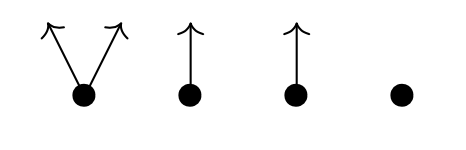If $M$ is an $m$-dimensional manifold, then you might view its tangent bundle $TM$ set-theoretically as the ‘corolla’ of the polynomial functor $M y^{\mathbb{R}^m}$, the tree-roots corresponding to the points of the manifold, and the branches to the different tangent vectors in these points.

Morphisms in $\mathbf{Poly}$ are a bit strange. For two polynomial functors $p=(p(1),p[-])$ and $q=(q(1),q[-])$ a map $p \rightarrow q$ in $\mathbf{Poly}$ consists of

• a map $\phi_1 : p(1) \rightarrow q(1)$ on the tree-roots in the right direction, and
• for any $i \in p(1)$ a map $q[\phi_1(i)] \rightarrow p[i]$ on the branches in the opposite direction

In our manifold/tangentbundle example, a morphism $My^{\mathbb{R}^m} \rightarrow y^1$ sends every point $p \in M$ to the unique root of $y^1$ and the unique branch in $y^1$ picks out a unique tangent-vector for every point of $M$. That is, vectorfields on $M$ are very special (smooth) morphisms $Mu^{\mathbb{R}^m} \rightarrow y^1$ in $\mathbf{Poly}$.

A smooth map between manifolds $M \rightarrow N$, does not determine a morphism $My^{\mathbb{R}^m} \rightarrow N y^{\mathbb{R}^n}$ in $\mathbf{Poly}$ because tangent vectors are pushed forward, not pulled back.

If instead we view the cotangent bundle $T^*M$ as the corolla of the polynomial functor $My^{\mathbb{R}^m}$, then everything works well.

But then, I promised not to use co-terminology…

Another time I hope to tell you how $\mathbf{Poly}$ helps us to understand the logic of learners.

Judging from this and that paper, deep learning is the string theory of the 2020s for geometers and representation theorists.

If you want to know quickly what neural networks really are, I can recommend the post demystifying deep learning.

The typical layout of a deep neural network has an input layer $L_0$ allowing you to feed $N_0$ numbers to the system (a vector $\vec{v_0} \in \mathbb{R}^{N_0}$), an output layer $L_p$ spitting $N_p$ numbers back (a vector $\vec{v_p} \in \mathbb{R}^{N_p}$), and $p-1$ hidden layers $L_1,\dots,L_{p-1}$ where all the magic happens. The hidden layer $L_i$ has $N_i$ virtual neurons, their states giving a vector $\vec{v_i} \in \mathbb{R}^{N_i}$.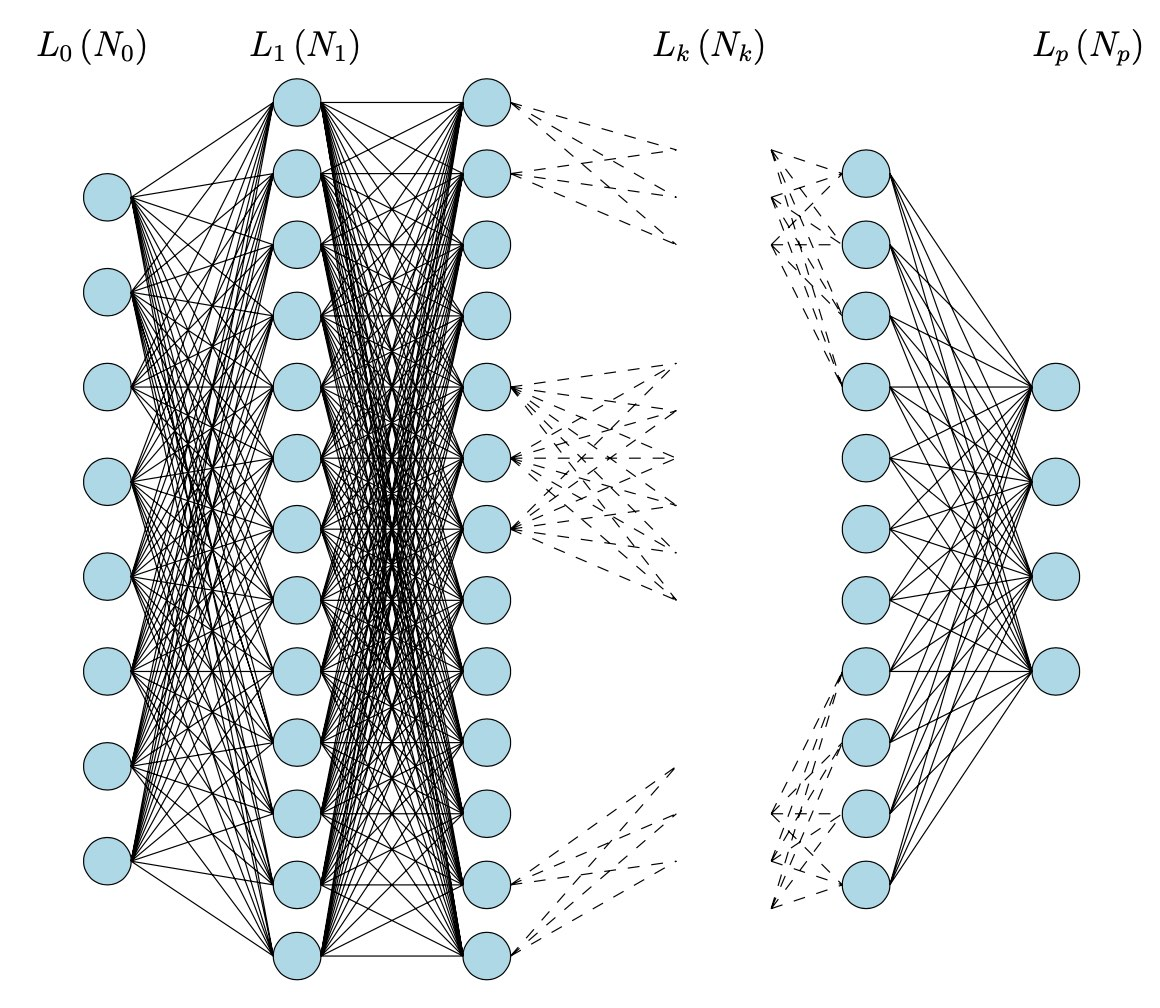Picture taken from Logical informations cells I

For simplicity let’s assume all neurons in layer $L_i$ are wired to every neuron in layer $L_{i+1}$, the relevance of these connections given by a matrix of weights $W_i \in M_{N_{i+1} \times N_i}(\mathbb{R})$.

If at any given moment the ‘state’ of the neural network is described by the state-vectors $\vec{v_1},\dots,\vec{v_{p-1}}$ and the weight-matrices $W_0,\dots,W_p$, then an input $\vec{v_0}$ will typically result in new states of the neurons in layer $L_1$ given by

$\vec{v_1}’ = c_0(W_0.\vec{v_0}+\vec{v_1})$

which will then give new states in layer $L_2$

$\vec{v_2}’ = c_1(W_1.\vec{v_1}’+\vec{v_2})$

and so on, rippling through the network, until we get as the output

$\vec{v_p} = c_{p-1}(W_{p-1}.\vec{v_{p-1}}’)$

where all the $c_i$ are fixed smooth activation functions $c_i : \mathbb{R}^{N_{i+1}} \rightarrow \mathbb{R}^{N_{i+1}}$.

This is just the dynamic, or forward working of the network.

The learning happens by comparing the computed output with the expected output, and working backwards through the network to alter slightly the state-vectors in all layers, and the weight-matrices between them. This process is called back-propagation, and involves the gradient descent procedure.

Even from this (over)simplified picture it seems doubtful that set valued (!) toposes are suitable to describe deep neural networks, as the Paris-Huawei-topos-team claims in their recent paper Topos and Stacks of Deep Neural Networks.

Still, there is a vast generalisation of neural networks: learners, developed by Brendan Fong, David Spivak and Remy Tuyeras in their paper Backprop as Functor: A compositional perspective on supervised learning (which btw is an excellent introduction for mathematicians to neural networks).

For any two sets $A$ and $B$, a learner $A \rightarrow B$ is a tuple $(P,I,U,R)$ where

• $P$ is a set, a parameter space of some functions from $A$ to $B$.
• $I$ is the interpretation map $I : P \times A \rightarrow B$ describing the functions in $P$.
• $U$ is the update map $U : P \times A \times B \rightarrow P$, part of the learning procedure. The idea is that $U(p,a,b)$ is a map which sends $a$ closer to $b$ than the map $p$ did.
• $R$ is the request map $R : P \times A \times B \rightarrow A$, the other part of the learning procedure. The idea is that the new element $R(p,a,b)=a’$ in $A$ is such that $p(a’)$ will be closer to $b$ than $p(a)$ was.

The request map is also crucial is defining the composition of two learners $A \rightarrow B$ and $B \rightarrow C$. $\mathbf{Learn}$ is the (symmetric, monoidal) category with objects all sets and morphisms equivalence classes of learners (defined in the natural way).

In this way we can view a deep neural network with $p$ layers as before to be the composition of $p$ learners
$\mathbb{R}^{N_0} \rightarrow \mathbb{R}^{N_1} \rightarrow \mathbb{R}^{N_2} \rightarrow \dots \rightarrow \mathbb{R}^{N_p}$
where the learner describing the transition from the $i$-th to the $i+1$-th layer is given by the equivalence class of data $(A_i,B_i,P_i,I_i,U_i,R_i)$ with
$A_i = \mathbb{R}^{N_i},~B_i = \mathbb{R}^{N_{i+1}},~P_i = M_{N_{i+1} \times N_i}(\mathbb{R}) \times \mathbb{R}^{N_{i+1}}$
and interpretation map for $p = (W_i,\vec{v}_{i+1}) \in P_i$
$I_i(p,\vec{v_i}) = c_i(W_i.\vec{v_i}+\vec{v}_{i+1})$
The update and request maps (encoding back-propagation and gradient-descent in this case) are explicitly given in theorem III.2 of the paper, and they behave functorial (whence the title of the paper).

More generally, we will now associate objects of a topos (actually just sheaves over a simple topological space) to a network op $p$ learners
$A_0 \rightarrow A_1 \rightarrow A_2 \rightarrow \dots \rightarrow A_p$
inspired by section I.2 of Topos and Stacks of Deep Neural Networks.

The underlying category will be the poset-category (the opposite of the ordering of the layers)
$0 \leftarrow 1 \leftarrow 2 \leftarrow \dots \leftarrow p$
The presheaf on a poset is a locale and in this case even the topos of sheaves on the topological space with $p+1$ nested open sets.
$X = U_0 \supseteq U_1 \supseteq U_2 \supseteq \dots \supseteq U_p = \emptyset$
If the learner $A_i \rightarrow A_{i+1}$ is (the equivalence class) of the tuple $(A_i,A_{i+1},P_i,I_i,U_i,R_i)$ we will now describe two sheaves $\mathcal{W}$ and $\mathcal{X}$ on the topological space $X$.

$\mathcal{W}$ has as sections $\Gamma(\mathcal{W},U_i) = \prod_{j=i}^{p-1} P_i$ and the obvious projection maps as the restriction maps.

$\mathcal{X}$ has as sections $\Gamma(\mathcal{X},U_i) = A_i \times \Gamma(\mathcal{W},U_i)$ and restriction map to the next smaller open
$\rho^i_{i+1}~:~\Gamma(\mathcal{X},U_i) \rightarrow \Gamma(\mathcal{X},U_{i+1}) \qquad (a_i,(p_i,p’)) \mapsto (p_i(a_i),p’)$
and other retriction maps by composition.

A major result in Topos and Stacks of Deep Neural Networks is that back-propagation is a natural transformation, that is, a sheaf-morphism $\mathcal{X} \rightarrow \mathcal{X}$.

In this general setting of layered learners we can always define a map on the sections of $\mathcal{X}$ (for every open $U_i$), $\Gamma(\mathcal{X},U_i) \rightarrow \Gamma(\mathcal{X},U_i)$
$(a_,(p_i,p’)) \mapsto (R(p_i,a_i,p_i(a_i)),(U(p_i,a_i,p_i(a_i)),p’)$
But, in order for this to define a sheaf-morphism, compatible with the restrictions, we will have to impose restrictions on the update and restriction maps of the learners, in general.

Still, in the special case of deep neural networks, this compatibility follows from the functoriality property of Backprop as Functor: A compositional perspective on supervised learning.

To be continued.

If Chad Orzel is able to teach quantum theory to his dog, surely it must be possible to explain schemes, stacks, toposes and motives to hipsters?

Perhaps an idea for a series of posts?

It’s early days yet. So far, I’ve only added the tag sga4hipsters (pun intended) and googled around for ‘real-life’ applications of sheaves, cohomology, and worse.

Sooner or later one ends up at David Spivak’s MIT-webpage.

David has written a book “category theory for scientists” and has several papers on applications of category theory to databases.

There’s also this hilarious abstract, reproduced below, of a talk he gave in 2007 at many cheerful facts.

If this guy ever decides to write a novel, I’ll pre-order it on the spot.Presheaf, the cobbler.
by David Spivak

Children have always loved colimits.

Whether it be sorting their blocks according to color, gluing a pair of googly eyes and a pipe-cleaner onto a piece of yellow construction paper, or simply eating a peanut butter sandwich, colimits play a huge role in their lives.

But what happens when their category doesn’t have enough colimits?

In today’s ”ownership” society, what usually happens is that the parents upgrade their child’s category to a Presheaf category. Then the child can cobble together crazy constructions to his heart’s content.

Sometimes, a kid comes up to you with an FM radio she built out of tinkertoys, and says
”look what I made! I call it ’182 transisters, 11 diodes, 6 plastic walls, 3 knobs,…’”

They seem to go on about the damn thing forever.

Luckily, Grothendieck put a stop to this madness.

He used to say to them, ever so gently, ”I’m sorry, kid. I’m really proud of you for making this ’182 transistors’ thing, but I’m afraid it already has a name. It’s called a radio.

And thus Grothendieck apologies were born.

Two years later, Grothendieck topologies were born of the same concept.

In this talk, I will teach you to build a radio (that really works!) using only a category of presheaves, and then I will tell you about the patent-police, known as Grothendieck topologies.

God willing, I will get through SGA 4 and Lurie’s book on Higher Topos Theory.”